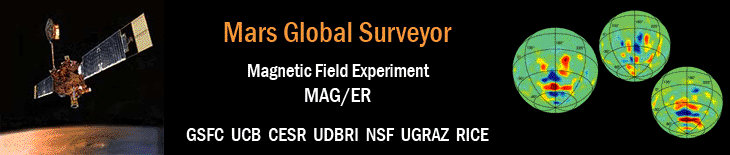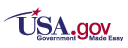'# The Global Magnetic Field of Mars and Implications for Crustal Evolution Map Data

## Connerney, J.E.P., M.H. Acuña, P.J. Wasilewski, G. Kletetschka, N.F. Ness, H. Rème, R.P. Lin, and D.L. Mitchell

Geophysical Research Letters, Vol. 28, Iss. 21, pp. 4015-4018, 1 Nov 2001.

For positions near the poles (latitudes between -86 degrees and -90 degress, and between 86 degrees and 90 degrees) the field values (Br, Bt, and Bp) may equal zero NT because the spacecraft's orbit does not cover those areas, so sampling was not possible.

## Mars Global Surveyor MAG/ER Maps - Ascii File

The file grl_28_connerney_map_data.txt contains the data available in the radial, theta, and phi maps in terms of B-field components in the order Br, Bt, Bp, and location of the spacecraft in the order r, latitude, and east longitude.

## Mars Global Surveyor MAG/ER Maps - IDL Files

The files
```br_360x180.bin or br_360x180_pc.bin (radial)
bt_360x180.bin or bt_360x180_pc.bin (theta)
bp_360x180.bin or bp_360x180_pc.bin (phi)
```
contain IDL unformatted data arrays of the maps of the radial (br), theta (bt), and phi (bp) components, respectively, of the vector magnetic field for Mars.

If the file(s) are to be read on a PC, please download the second file (i.e., "_pc" in the name) for each component.

The following IDL commands will allow the user to read the files:

```IDL> br = fltarr(360,180)
IDL> openr, lun, 'br_360x180.bin', /get_lun
IDL> free_lun, lun

IDL> bt = fltarr(360,180)
IDL> openr, lun, 'bt_360x180.bin', /get_lun
IDL> free_lun, lun

IDL> bp = fltarr(360,180)
IDL> openr, lun, 'bp_360x180.bin', /get_lun
IDL> free_lun, lun
```
Each value in the data array represents the median for all data within an 1 degree by 1 degree bin. The arrays are arranged with the first index equal to the minimum east longitude in degrees - ranging from 0 degrees to 359 degrees - of the bin. The second index is related to the latitude where index 0 is the bin from -90 degrees to and including -89 degrees and index 179 is the bin from +89 degrees to and including +90 degrees (i.e., second index = minimum latitude + 90).

A couple examples are

• for longitude bin 171 degrees through 172 degrees, latitude bin -55 degrees through -54 degrees,
center longitude is 171.5 degrees and center latitude is -54.5 degrees, and
data would be at the indices (171,35) with br(171,35) = 138.271 nT, bt(171,35) = 79.2177 nT, and bp(171,35) = -50.1592 nT

• for longitude bin 200 degrees through 201 degrees, latitude bin 45 degrees through 46 degrees,
center longitude is 200.5 degrees and center latitude is 45.5 degrees, and
data would be at the indices (200,135) with br(200,135) = -7.36658 nT, bt(200,135) = -3.72621 nT, and bp(200,135) = 5.03452 nT

To obtain an image similar to figure 1, it is necessary to first set minimum and maximum values such that they are symmetric about 0. For figure 1 (radial component), one element is set to a negative value equal to the maximum positive value to insure that the center of the colorbar is at zero field.

```IDL> print, min(br), max(br)
-130.005      221.079
IDL> br[0,0] = -221.
IDL> tvscl, br

(use xloadct to select Blue-Red colorbar and 20%-80% stretch)

```